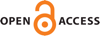#### Vol. 8, No. 1, 2018

 Download this articleFor screen For printingRecent Issues Volume 11, Issue 1 Volume 10, Issue 1 Volume 9, Issue 2 Volume 9, Issue 1 Volume 8, Issue 1 Volume 7, Issue 1 Volume 6, Issue 1 Volume 5, Issue 1 Volume 4, Issue 1 Volume 3, Issue 1 Volume 2, Issue 1 Volume 1, Issue 1The Journal About the Journal Editorial Board Submission Guidelines Submission Form Policies for Authors Ethics Statement ISSN: 1948-7916 Author Index To Appear Other MSP JournalsExteriorIdeals: a package for computing monomial ideals in an exterior algebra

### Luca Amata and Marilena Crupi

Vol. 8 (2018), 71–79##### Abstract

Let $K$ be a field, $V$ a $K$-vector space with basis ${e}_{1},\dots ,{e}_{n}$, and $E$ the exterior algebra of $V$. We introduce a Macaulay2 package that allows one to deal with classes of monomial ideals in $E$. More precisely, we implement in Macaulay2 some algorithms in order to easily compute stable, strongly stable and lexsegment ideals in $E$. Moreover, an algorithm to check whether an $\left(n+1\right)$-tuple $\left(1,{h}_{1},\dots ,{h}_{n}\right)$ (${h}_{1}\le n={dim}_{K}V$) of nonnegative integers is the Hilbert function of a graded $K$-algebra of the form $E∕I$, with $I$ a graded ideal of $E$, is given. In particular, if ${H}_{E∕I}$ is the Hilbert function of a graded $K$-algebra $E∕I$, the package is able to construct the unique lexsegment ideal ${I}^{lex}$ such that ${H}_{E∕I}={H}_{E∕{I}^{lex}}$.

##### Keywords
exterior algebra, monomial ideals, Hilbert functions, algorithms
##### Mathematical Subject Classification 2010
Primary: 13A02, 15A75, 68W30
##### Supplementary material

ExteriorIdeals.m2 - Macaulay2 package for computing monomial ideals in an exterior algebra